# Division with Fractions

Reduce the numbers to simple fractions. Invert the divisor and proceed as in multiplication. If the quantities are mixed numbers, multiply both numbers by the fractional part of the divisor and divide as in division of integers; and if a mixed fraction remains, reduce it to a simple one.

Examples:

(1) Divide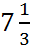by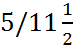.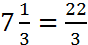and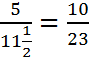. Therefore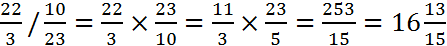.

(2) Divide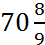by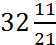.

As a first step multiply both numbers by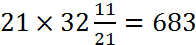: and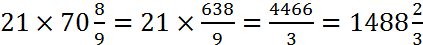.

Now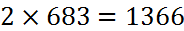so that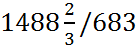is 2 with a remainder of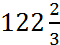or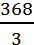. Therefore the final answer is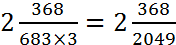.